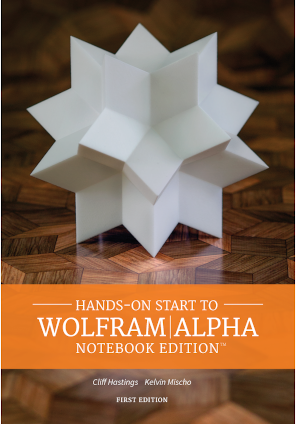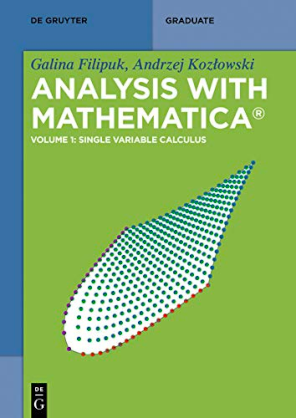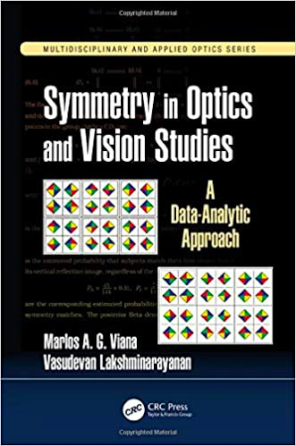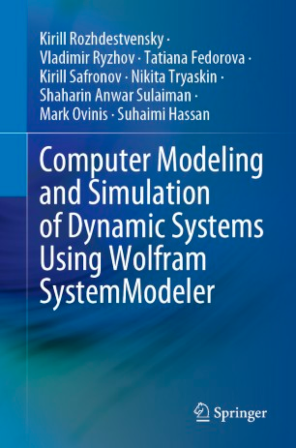Browse by Topic
Related Topics

# New Wolfram Language Books on Wolfram|Alpha, Calculus, Applied Engineering and System Modeler

The pandemic has postponed or canceled a lot of things this year, but luckily learning isn’t one of them. Check out these picks for new Wolfram Language books that will help you explore new software, calculus, engineering and more from the comfort of home.

## Hands-on Start to Wolfram|Alpha Notebook EditionNew from Wolfram Media and the authors of Hands-on Start to Wolfram Mathematica, Hands-on Start to Wolfram Alpha|Notebook Edition is now available! Wolfram|Alpha Notebook Edition combines the simplicity of Wolfram|Alpha with the computational capabilities of Mathematica for the best of both in a single, unified tool perfect for teaching and learning. Use free-form input to get instant answers to questions, create and customize graphs, and turn static examples into dynamic models. Everything is saved as an interactive Wolfram Notebook, so you can add notes and use notebooks as class or reference materials or present them as dynamic slide shows that engage your audience as you edit examples on the fly.

With this book, you’ll learn how to:

• Quickly create notebooks that combine calculations, graphics, interactive examples and notes.
• Enter free-form input and get solutions for a variety of calculations (e.g. arithmetic, algebra, calculus, linear algebra).
• Access step-by-step solutions, suggestions for next steps and related computations.
• Create 2D, 3D and interactive graphics with controls to dynamically change the parameters.
• Use previous results in future calculations, assign variables and define functions.
• Create dynamic slide show presentations with interactive elements that can be changed on the fly.

## Analysis with Mathematica: Volume 1: Single Variable CalculusIn this De Gruyter textbook, Galina Filipuk and Andrzej Kozłowski explore how Mathematica is able to do much more than just numerics. This text shows how to tackle real mathematical problems from basic analysis. Readers will learn how Mathematica represents domains, qualifiers and limits to implement actual proofs—a requirement to unlock the huge potential of Mathematica for a variety of applications. While this volume focuses on single variable calculus, readers should also keep an eye out for Volume 2: Multi-variable Calculus, which is scheduled to be published in 2021.

## Symmetry in Optics and Vision Studies: A Data-Analytic ApproachIn this book, authors Marlos A. G. Viana and Vasudevan Lakshminarayanan present an introduction to the foundations, interpretations and data-analytic applications of symmetry studies with an emphasis on applications in optical sciences. Symmetry studies connect group theoretic and statistical methods for data summary and inference. This book is written for an audience familiar with calculus and linear algebra as well as introductory statistics. The book reviews finite group theory in the introductory chapters. Computational tools used in the text are also available for download as Mathematica notebooks, offering readers an interactive experience.

## Advanced Engineering Mathematics with MathematicaAdvanced Engineering Mathematics with Mathematica presents advanced analytical solution methods that are used to solve boundary value problems in engineering and integrates these methods with Mathematica procedures. In this book, author Edward B. Magrab emphasizes the Sturm–Liouville system and the generation and application of orthogonal functions, which are used by the separation of variables method to solve partial differential equations. It introduces the relevant aspects of complex variables, matrices and determinants, Fourier series and transforms, solution techniques for ordinary differential equations, the Laplace transform and procedures to make ordinary and partial differential equations used in engineering non-dimensional. Magrab presents numerous and widely varied solved boundary value problems to show the diverse applications of the material.

## Computer Modeling and Simulation of Dynamic Systems Using Wolfram SystemModelerGeared toward students and professionals in the field of dynamic system modeling, this book briefly discusses the main provisions of the theory of modeling. It also describes in detail the methodology for constructing computer models of dynamic systems using the Wolfram visual modeling environment, System Modeler, and provides illustrative examples of solving problems of mechanics and hydraulics. This book serves as a supplement to university courses in modeling and simulation of dynamic systems, but it’s also a great resource for anyone looking for examples and explanations of System Modeler.

## Applied Engineering MathematicsThis textbook is written for undergraduate engineering students (specifically those in years 2–4 of an engineering degree course) and it places a strong emphasis on visualization and the methods and tools needed across the whole of engineering. Author Brian Vick emphasizes the visual approach while avoiding excessive proofs and derivations. The visual images explain and teach the mathematical methods. The book’s website provides Mathematica notebooks with dynamic and interactive code to accompany the examples for the reader to explore with Mathematica or the free Wolfram Player, and it provides access for instructors to a solutions manual.

If you’re interested in finding more books that use the Wolfram Language, check out the full collection on the Wolfram Books site. If you’re working on a Mathematica or Wolfram Language book, contact us to find out more about our options for author support and to have your book featured in an upcoming blog post!

 Get full access to the latest Wolfram Language functionality with a Mathematica 12.2 or Wolfram|One trial.